# Uncertainty principle, mathematical

The following meta-theorem: It is not possible for a non-trivial function and its Fourier transform to be simultaneously sharply localized/concentrated.

Depending on the definition of the term "concentration" , one gets various concrete manifestations of this principle, one of them (see the Heisenberg uncertainty inequality below), correctly interpreted, is in fact the celebrated Heisenberg uncertainty principle of quantum of mechanics in disguise ([a13]).

A comprehensive discussion of various (mathematical) uncertainty principles can be found in [a10].

## Heisenberg uncertainty inequality.

Defining concentration in terms of standard deviation leads to the Heisenberg uncertainty inequality. Ifand, the quantity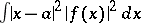is a measure of the concentration ofaround. Roughly speaking, the more concentratedis around, the smaller will this quantity be. If one normalizessuch that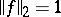, then by the Plancherel theorem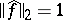. Here,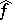is the Fourier transform of, defined bythe convergence of the integral being interpreted suitably. Then, for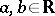one has the Heisenberg inequality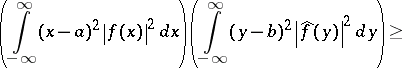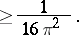Thus, the above says that ifis concentrated around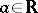, then no matter what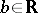is chosen,cannot be concentrated around. Equality is attained in the above if and only ifis, modulo translation and multiplication by a phase factor, a Gaussian function (i.e. of the form).

## Benedicks' theorem.

Concentration can also be measured in terms of the "size" of the set on whichis supported (cf. also Support of a function). If one takes "size" to mean Lebesgue measure, then M. Benedicks ([a4], [a1]) has proved the following result: Ifis a non-zero function, then it is impossible for bothand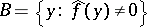to have finite Lebesgue measure. (This is a significant generalization of the fact, well known to communication engineers, that a function cannot be both time limited and band limited.) For various other uncertainty principles of this kind, see [a11].

## Hardy's uncertainty principle.

Another natural way of measuring concentration is to consider the rate of decay of the function at infinity. A result of G.H. Hardy [a12] states that bothandcannot be simultaneously "very rapidly decreasing" . More precisely: If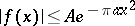,for some positive constants,,,and for all, and if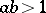, then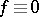. (If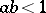, then there are infinitely many linearly independent functionssatisfying the inequalities, and if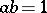, thenmust be necessarily a Gaussian function.) Actually, the first part of Hardy's result can be deduced from the following more general result of A. Beurling [a14]: If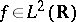is such that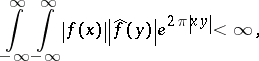then. There are various refinements of Hardy's theorem (see [a6] for one such refinement).

## Other directions.

Apart from the three instances of the mathematical uncertainty principle described above, there are a host of uncertainty principles associated with different ways of measuring concentration (see, e.g., [a2], [a3], [a5], [a7], [a8], [a9], [a15], [a16], [a18], [a19]).

Ifis a locally compact group (including the case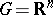), then it is possible to develop a Fourier transform theory for functions defined on(cf. also Harmonic analysis, abstract). There is a considerable body of literature devoted to deriving various uncertainty principles in this context also. (See the bibliography in [a10].)

The Fourier inversion formula can be thought of as an eigenfunction expansion with respect to the standard Laplacian (cf. also Laplace operator; Eigen function). So it is natural to seek uncertainty principles associated with other eigenfunction expansions. Although this has not been as systematically developed as in the case of standard Fourier transform theory, there are several results in this direction as well (see [a17] and the bibliography in [a10]).